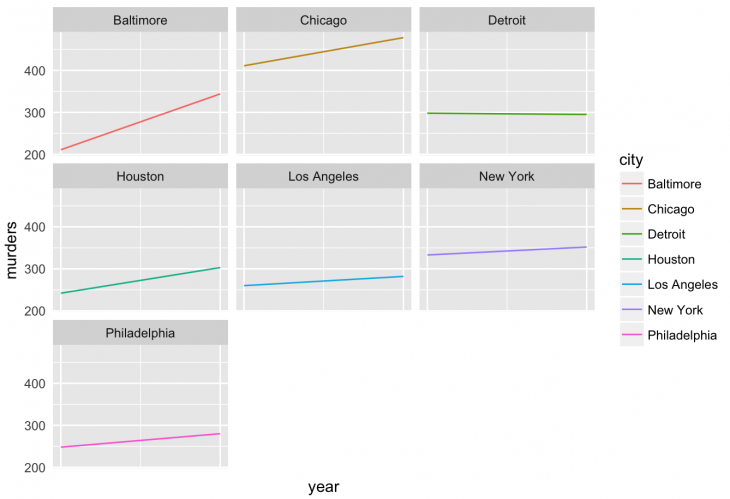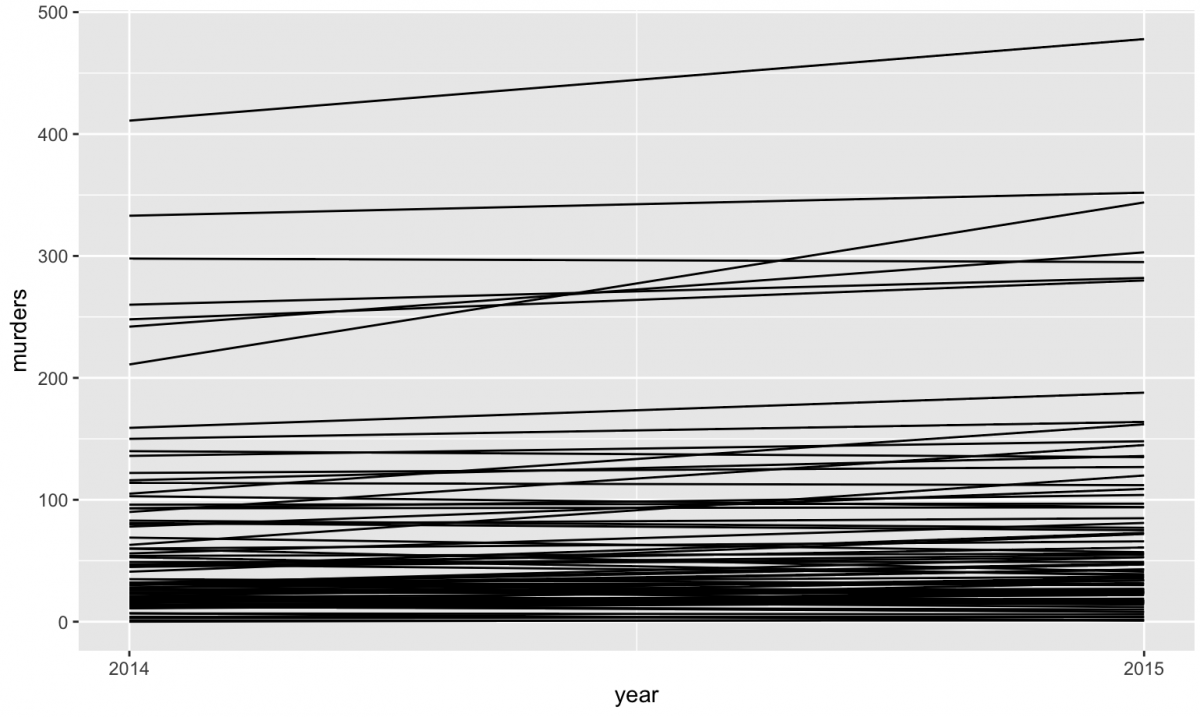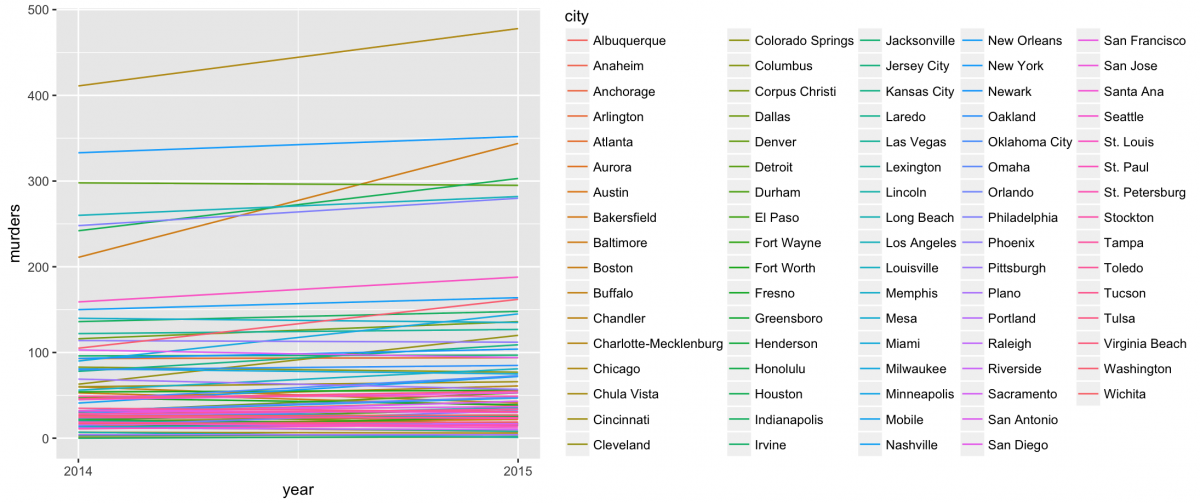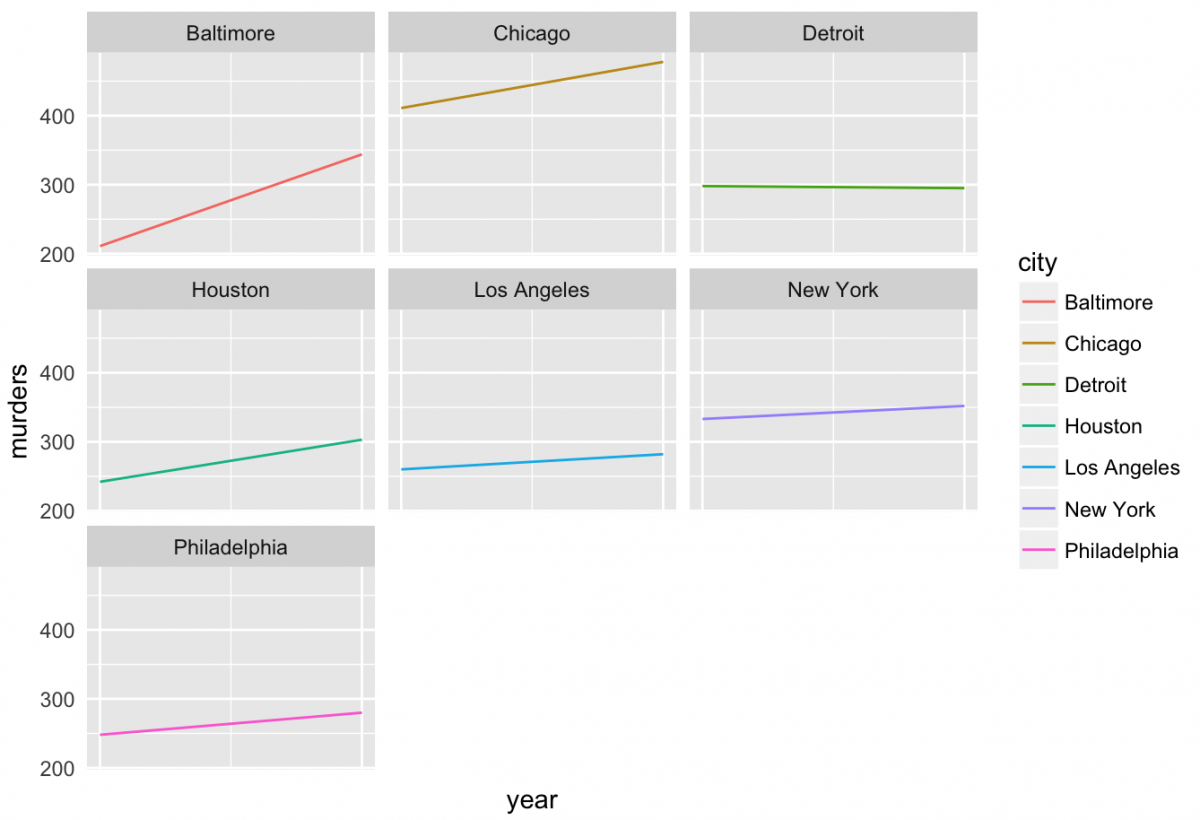# How to make small multiples in R using geom_line()

We’ve been exploring and visualizing datasets from the `fivethirtyeight` R package for various Storybench tutorials. (See our tidyr tutorial and our barplot tutorial). Below, we’ve written a tutorial to create a grid of line charts that all use the same scale and axes in R – otherwise known as small multiples.

Small multiples are a popular choice for visualizing a series of graphs to allow easy comparison. FiveThirtyEight is fond of using them.The following tutorial uses data about 2014 and 2015 murders in U.S. cities from the `murder_2015_final` dataset found in FiveThirtyEight’s R package. The tutorial uses murders_separate.csv, which was cleaned and created in this Storybench tutorial.

If you’re new to R, start here. If you’re new to data visualization in R, start here.

## Load ggplot2 and the csv

Load `library(ggplot2)` and then the csv with:

``````library(ggplot2)

## View the dataset in RStudio

Once you’ve called the dataset, you can view its column names with `names(murders_separate)` or see the data table in RStudio using `View(murders_separate)`. Note the capital V in View.## Plot all cities to see change in murders between 2014 and 2015

Using `ggplot()`, call on the `murders_separate` dataset and then create a line chart plotting `year` on the x-axis and `murders` on the y-axis. By grouping by `city`, ggplot will create one line for each city. Next, add a plus sign (`+`) and then `geom_line()` to draw the chart. Adding `scale_x_continuous(breaks=c(2014,2015))` will remove the extraneous ticks – like 2014.25 and 2014.50 – and just mark 2014 and 2015 on the x-axis.

``````ggplot(murders_separate, aes(x=year, y=murders, group=city)) +
geom_line() +
scale_x_continuous(breaks=c(2014,2015))``````
``` ```## Add color and a legend

Add `color=city` to add a legend and color to each of the lines.

``````ggplot(murders_separate, aes(x=year, y=murders, group=city, color=city)) +
geom_line()+
scale_x_continuous(breaks=c(2014,2015))``````
``` ```This is a pretty busy graphic, so why don't we limit the dataset to only cities with at least 150 murders?

## Plotting only the 10 cities with over 150 murders

Using `subset` is one way to constrain the dataset. We've used `ggplot(subset(murders_separate, murders > 150)` in the following way:

``````ggplot(subset(murders_separate, murders > 150), aes(x=year, y=murders, group=city, color=city)) +geom_line()+
scale_x_continuous(breaks=c(2014,2015))``````
``` ```

That will produce the following chart:## Using facet_grid() to stack line plots

Now, to start making small multiples. `ggplot's facet_grid()`, documentation here, will split a plot into individual panels. By using `facet_grid(city ~ .)`, we can stack the seven cities with over 200 murders.

``````ggplot(subset(murders_separate, murders > 200), aes(x=year, y=murders, group=city, color=city)) +geom_line()+
facet_grid(city ~ .)+
scale_x_continuous(breaks=c(2014,2015))``````
``` ```## Create small multiples with cities with murders over 200

Finally, we can use `facet_wrap(~ city)` to split these into a matrix of small multiples.

``````ggplot(subset(murders_separate, murders > 200), aes(x=year, y=murders, group=city, color=city)) +geom_line()+
facet_wrap(~ city)+
scale_x_continuous(breaks=c(2014,2015))``````
``` ```

To add a title, use `+ labs(title = "Murder change between 2014 and 2015")`. If you want to remove the legend and 2014 and 2015 labels, use `+ theme(axis.ticks = element_blank(), axis.text.x = element_blank(), legend.position = "none")`.

``` ```
``````ggplot(subset(murders_separate, murders > 200), aes(x=year, y=murders, group=city, color=city)) +
geom_line()+
facet_wrap(~ city)+
labs(title = "Murder change between 2014 and 2015")+
scale_x_continuous(breaks=c(2014,2015))+
theme(axis.ticks = element_blank(), axis.text.x = element_blank(), legend.position = "none")``````## The full script